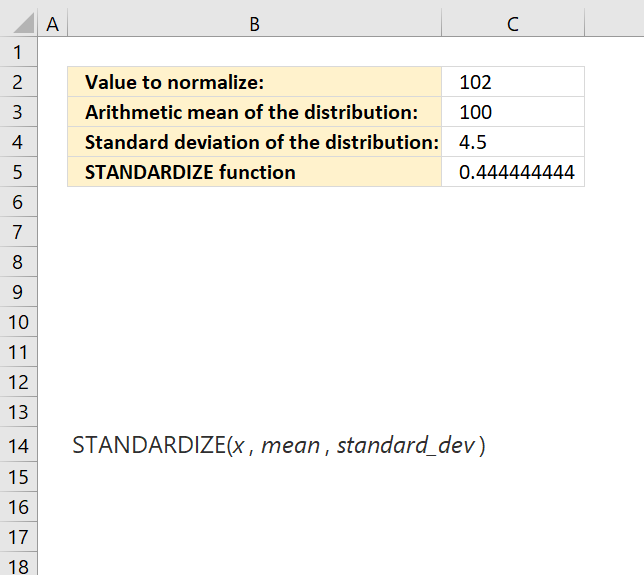Author: Oscar Cronquist Article last updated on May 11, 2022The STANDARDIZE function calculates a normalized value from a distribution characterized by mean and standard_dev.

Formula in cell C5:

=STANDARDIZE(C2, C3, C4)

### Excel Function Syntax

STANDARDIZE(x, mean, standard_dev)

### Arguments

 x Required. The value you want to normalize. Mean Required. The arithmetic mean of the distribution. Standard_dev Required. The standard deviation of the distribution.